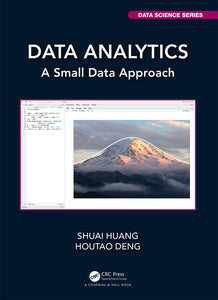# Data Analytics : A Small Data Approach

Regular price \$89.95 \$89.95 Sale

Author(s): Shuai Huang, Houtao Deng

Data Analytics: A Small Data Approach is suitable for an introductory data analytics course to help students understand some main statistical learning models. It has many small datasets to guide students to work out pencil solutions of the models and then compare with results obtained from established R packages. Also, as data science practice is a process that should be told as a story, in this book there are many course materials about exploratory data analysis, residual analysis, and flowcharts to develop and validate models and data pipelines.

The main models covered in this book include linear regression, logistic regression, tree models and random forests, ensemble learning, sparse learning, principal component analysis, kernel methods including the support vector machine and kernel regression, and deep learning. Each chapter introduces two or three techniques. For each technique, the book highlights the intuition and rationale first, then shows how mathematics is used to articulate the intuition and formulate the learning problem. R is used to implement the techniques on both simulated and real-world dataset. Python code is also available at the book’s website: http://dataanalyticsbook.info.

Review(s):

EAN: 9781000372458